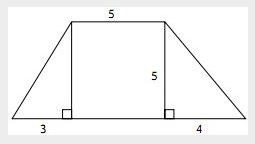# The area of the figure is42.5

Explanation

Add the areas of the two triangles and the square to find the total area. The area of the square is 52 = 25. Both triangles have a height of 5. The area of one triangle is $$\frac{1}{2} \times b \times h=\frac{1}{2} \times 3 \times 5=\frac{15}{2}=7.5$$. The area of the other triangle is $$\frac{1}{2} \times b \times h=\frac{1}{2} \times 4 \times 5=\frac{20}{2}=10$$. The total area is $$25+7.5+10 = 42.5$$.